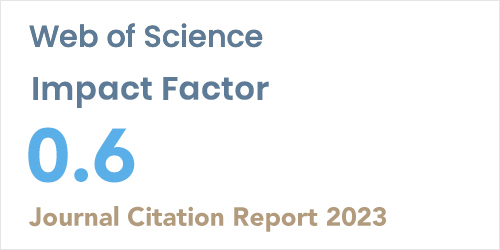ISSN: 1304-7191 | E-ISSN: 1304-7205
Volume: 41 Issue: 4 Year: 2023Estimation of Censored Regression Model in the Case of Non-Normal Error
1Department of Statistics, Anadolu University, ESKISEHİR
Sigma J Eng Nat Sci 2018; 36(2): 513-521

#### Abstract

For the censored regression model, it is well-known that while classical least squares estimation yields biased and nonconsistent estimator, maximum likelihood estimator (MLE) is consistent and efficient. Tobit estimator (Tobit model) based on MLE of normal error distribution is commonly-used estimation method for estimating censored regression in econometric literature. However, while the Tobit estimator works well for normal error distribution, its estimates may be inefficient in the case of non-normal errors. To solve this problem, different error distributions for the censored regression model have been proposed and tested in the literature. In this study, we consider the censored regression model based on the generalized logistic distribution. Generalized logistic distribution is very flexible distribution and approximates normal distribution for the special parameter cases. The considered estimator for the censored regression is evaluated by means of a simulation study designed in different combination of various error distributions and sample sizes. The results of the simulation show that the estimator of the censored regression model based on the generalized logistic distribution provides good performance for different error distributions and it is particularly good for small sample sizes. Moreover, when it is compared to classical Tobit estimator, efficiency loss of the considered estimator is very small for normal error distribution.